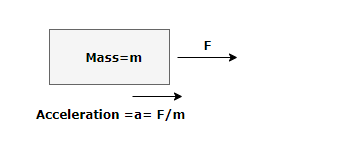# Force Calculator

Note
• Enter the values of the two known variables in the text boxes
• Leave the text box empty for the variable you want to solve for
• Click on the calculate button.

Formula used
$F=ma$

## What is Force

Force is a push or pull exerted on the body. We can also define force as an influence causing a body at rest or moving with constant velocity to undergo an accleration. As per Newton's second law
The net force on a body is equal to the product of mass and acceleration of the body
$F=ma$
where
F-> Net Force Acting on the Body
m -> Mass of the body
a -> Acceleration of the body because of the force
Force is a vector quantity .It has both magnitude and direction. The SI unit of Force is Newton
Example of Few questions where you can use this Force Calculator
Question 1
A Body of mass 4 kg is moving with the acceleration 2 m/s2.Find the force acting on it
Solution
m = 4 kg, a =2 m/s2
Now as per force formula
$f=ma = 4 \times 2 = 8 \ N$

Question 2
A Force of 10 N acts on the body of mass 2 kg.Find the acceleration of the body.
Solution
m = 2 kg, a =? , F=10 N
Now as per force formula
$f=ma$
or
$a = \frac {F}{m} = \frac {10}{2} = 5 \ m/s^2$

Question 3
A Force of 50 N acts on the body and the acceleration of the body is 20m/s2 .Find the mass of the body
Solution
m = ?, a =20m/s2, F=50 N
Now as per force formula
$f=ma$
or
$m = \frac {F}{a} = \frac {50}{20} = 2.5 \ kg$

## How the Force Calculator works1. If mass and acceleration are given, Force is calculated as
$F= ma$
2. If mass and force are given, acceleration is calculated as
$a= \frac {F}{m}$
3. If acceleration and force are given, mass is calculated as
$m = \frac {F}{a}$